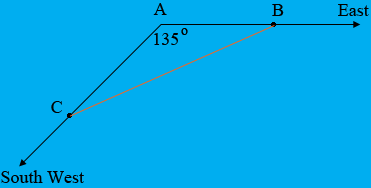SEARCH HOMEMath Central Quandaries & QueriesQuestion from Halley, a student: Two boats leave port at the same time. Boat A travels east at a speed of 12 km/hr. Boat B travels southwest at a speed of 14 km/hr. After two hours, how far apart are the boats? North is 0 degrees. How do I figure this out. ThanksHi Halley,

Did you draw a diagram? Here is mine.The two boats start at $A.$ The first travels east for 2 hours at 12 km/h to the point $B.$ what is the distance from $A$ to $B?$ The second boat travels south west for 2 hours at 14 km/hr to the point $C.$ What is the distance from $A$ to $C?$ The measure of the angle $BAC$ is $90^o + 45^o = 135^{o}.$

Thus you have a triangle, you know the lengths of two of the sides, the measure of the angle between these sides, and you want to know the length of the third side. This problem is taylor-made for the Law of Cosines.

PennyMath Central is supported by the University of Regina and the Imperial Oil Foundation.